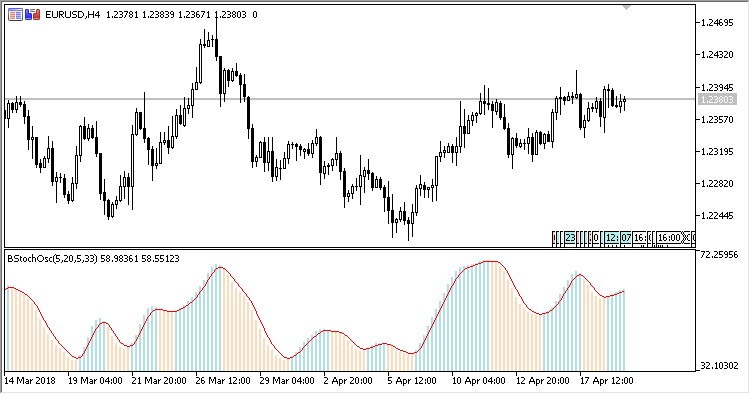# Blau_TS_Stochastic – indicator for MetaTrader 5

• A+
category：MT5 INDICATORS

The William Blau Stochastic Oscillator indicator shows the William Blau stochastic oscillator.

It has six input parameters:

• Period - calculation period;
• First smooth period - the initial smoothing period;
• Second smooth period - the secondary smoothing period;
• Third smooth period - the resulting smoothing period;
• Signal period - the signal line period;
• Applied price - price used for calculations.

Calculations:

```Blau_TStoch = 100*Stoch_EMA3/HH_EMA3,
Signal = EMA(Blau_TStoch, Signal period)
```

where:

```Stoch_EMA3 = EMA(EMA(EMA(Stoch, First smooth period), Second smooth period), Third smooth period),
HH_EMA3 = EMA(EMA(EMA(HH, First smooth period), Second smooth period), Third smooth period),
Stoch [i] = Price[i]-Min,
HH[i] = Max-Min,
Max, Min are the highest and the lowest price within the range from (i-Period+1) to (i)
```